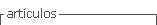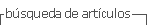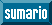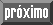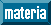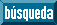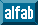## Articulo

•Citado por SciELO
•Accesos

•Similares en SciELO

## versión On-line ISSN 2528-7907versión impresa ISSN 1390-7247

#### Resumen

BARAHONA AVECILLA, Fredy; MUY CABRERA, Nelson; BARRERA CARDENAS, Olga  y  CARRETERO POBLETE, Pedro A. Mathematical modulation in the urban form of the canton of Riobamba (Ecuador). Revista San Gregorio [online]. 2020, n.43, pp.210-227. ISSN 2528-7907.  https://doi.org/10.36097/rsan.v1i43.1529.

The initial problem is the disorderly growth of the city of Riobamba (horizontal and irregular tendency), since it does not present characteristics of modular order, for which a projection was made to the years 2040 and 2100, in order to visualize the possibilities of suggesting corrections that restrain urban irregularities. To explain these modular processes, statistical information concerning the city is merged with the application of mathematical formulas (equations), which show numerically which geometries (perimeters, areas) are suitable for an urban planning process. The results are given on the basis of the study of regular polygons with the following (n) possibilities (n=∞; n=6; n=5; n=4; n=3), which make it possible to obtain projections of resource losses in construction and recommendations on optimal types and sizes. With the conclusion that, in the growth of the city of Riobamba until 2014 would have needed between 22.26 km and 28.62 km, instead of the 60,027 km, which required the current irregular form. And it turned out that the most appropriate geometric shape for the growth of the city would be the circle, since the indiscriminate growth of the city will pose problems in the environment in the future.

Palabras clave : intermediate cities; mathematical modulation; periphery; optimal perimeters; geometric projection.

· resumen en Español     · texto en Español     · Español (pdf )